# RS Aggarwal Solutions for Class 9 Chapter 17: Bar Graph, Histogram and Frequency Polygon Exercise 17B

## RS Aggarwal Solutions for Class 9 Maths Exercise 17B PDF

The main aim of the subject experts at BYJU’S is to provide questions and answers which are based on the latest CBSE syllabus. The solutions can be referred by the students to score well in the board exams. The students can enhance their problem solving abilities by using RS Aggarwal Solutions for Class 9. All the exercise wise problems are made available which can be downloaded for free by the students. The students can obtain a better hold on concepts with the help of solutions created by our faculties. RS Aggarwal Solutions for Class 9 Maths Chapter 17 Bar Graph, Histogram and Frequency Polygon Exercise 17B are provided here.

## RS Aggarwal Solutions for Class 9 Chapter 17: Bar Graph, Histogram and Frequency Polygon Exercise 17B Download PDF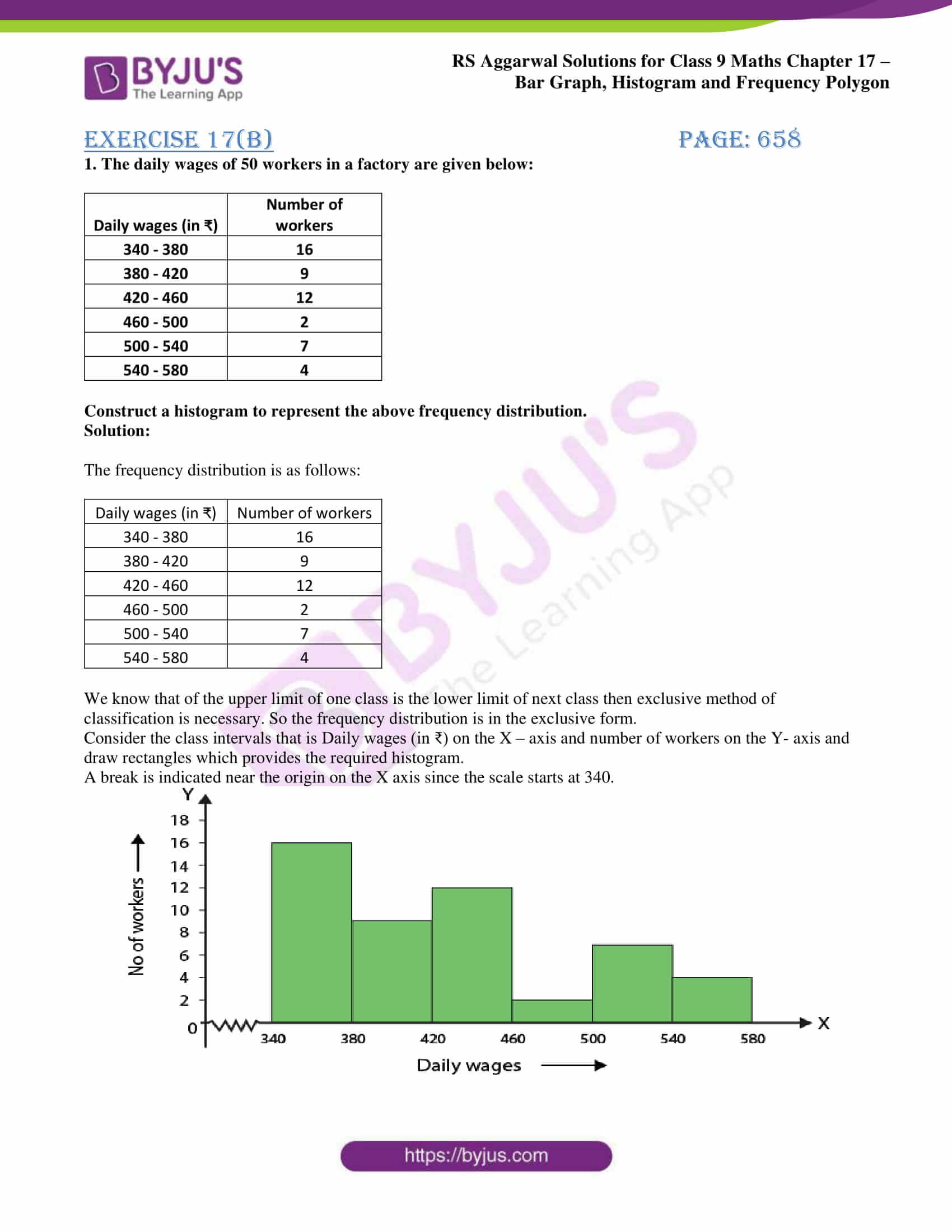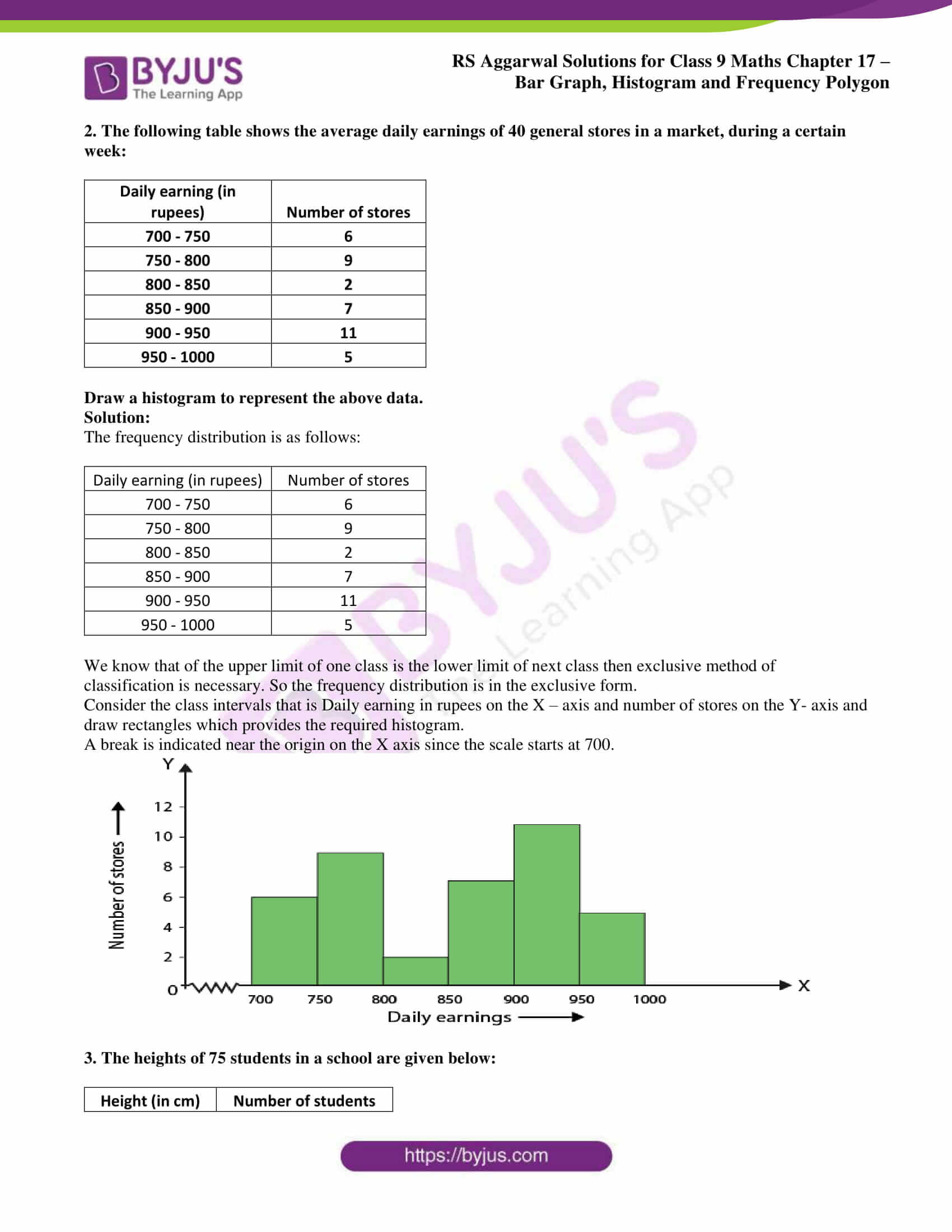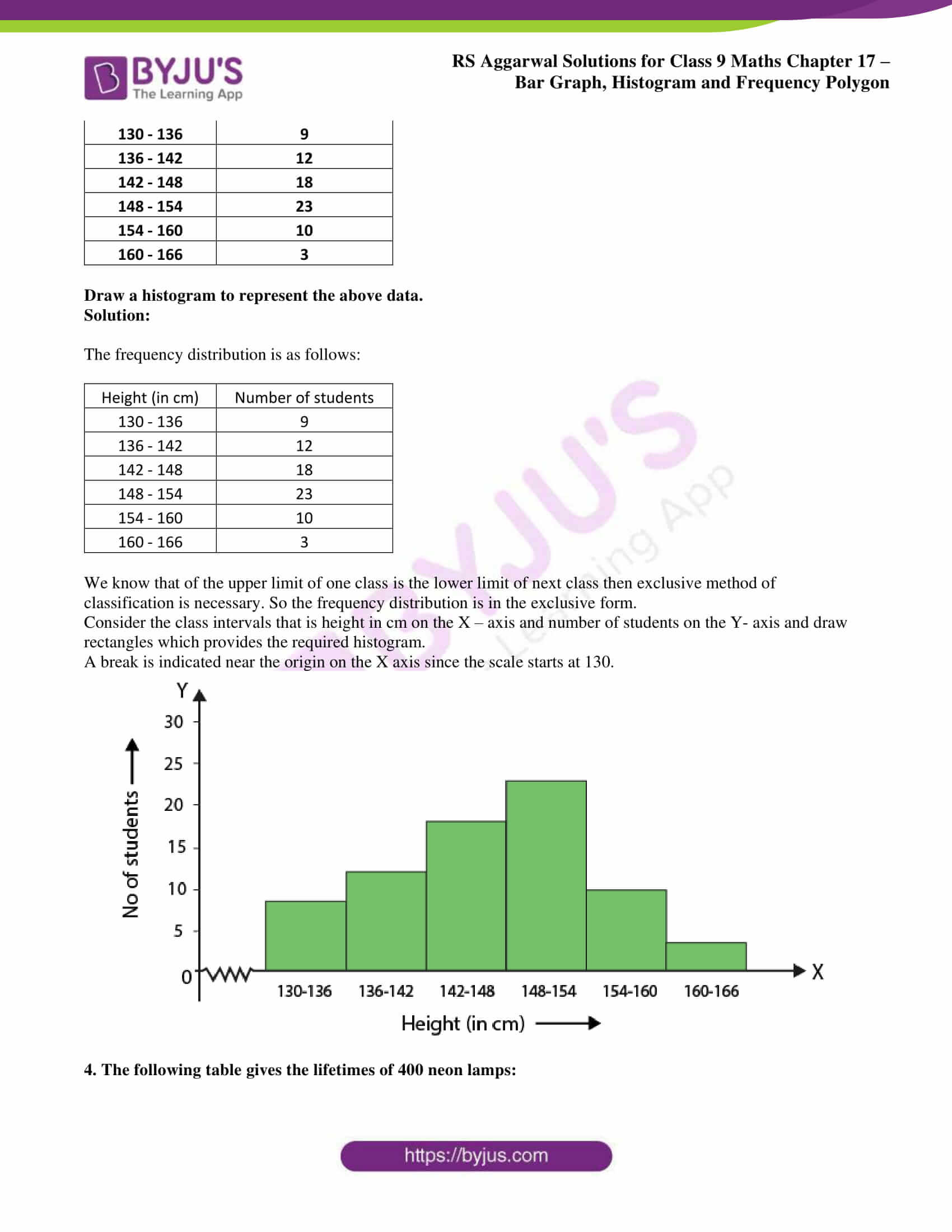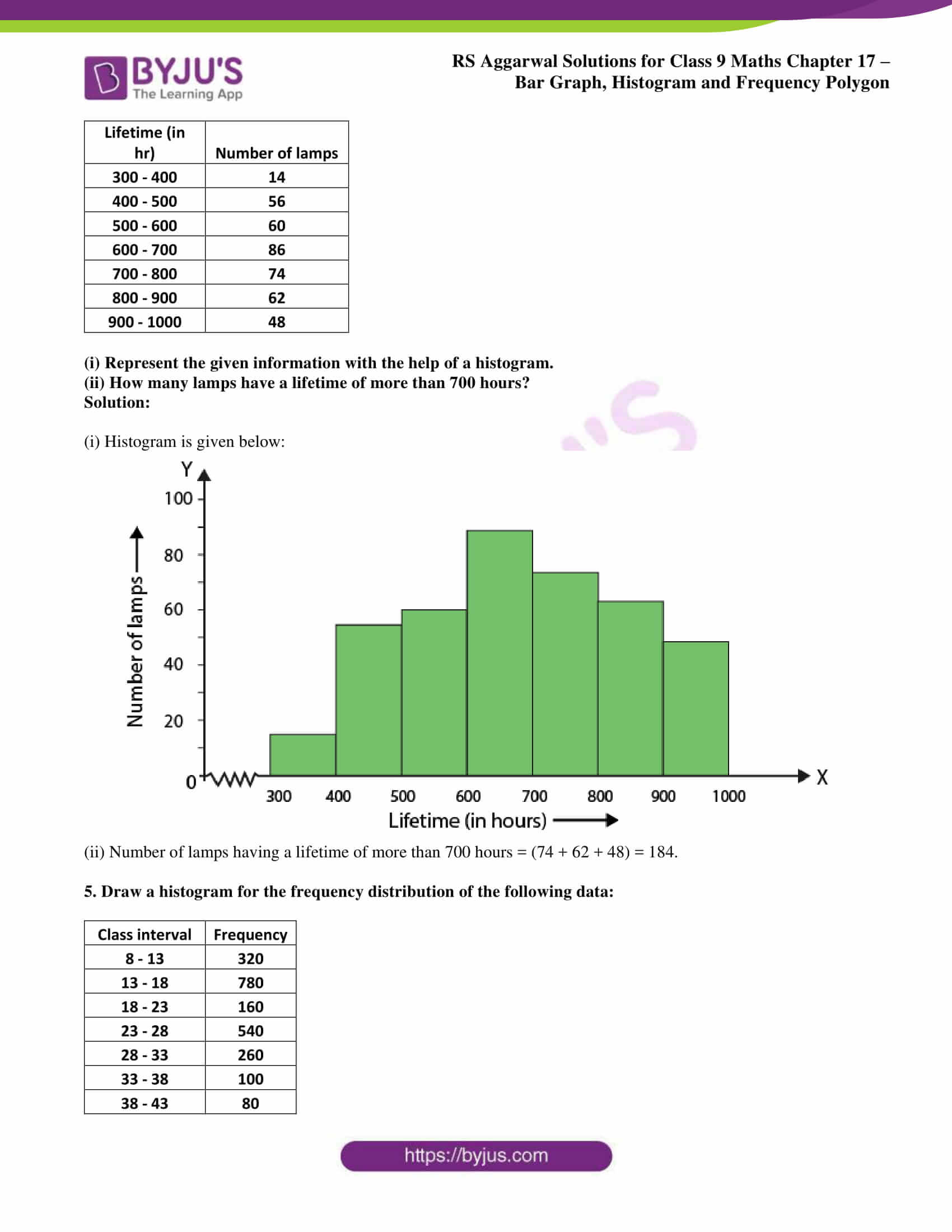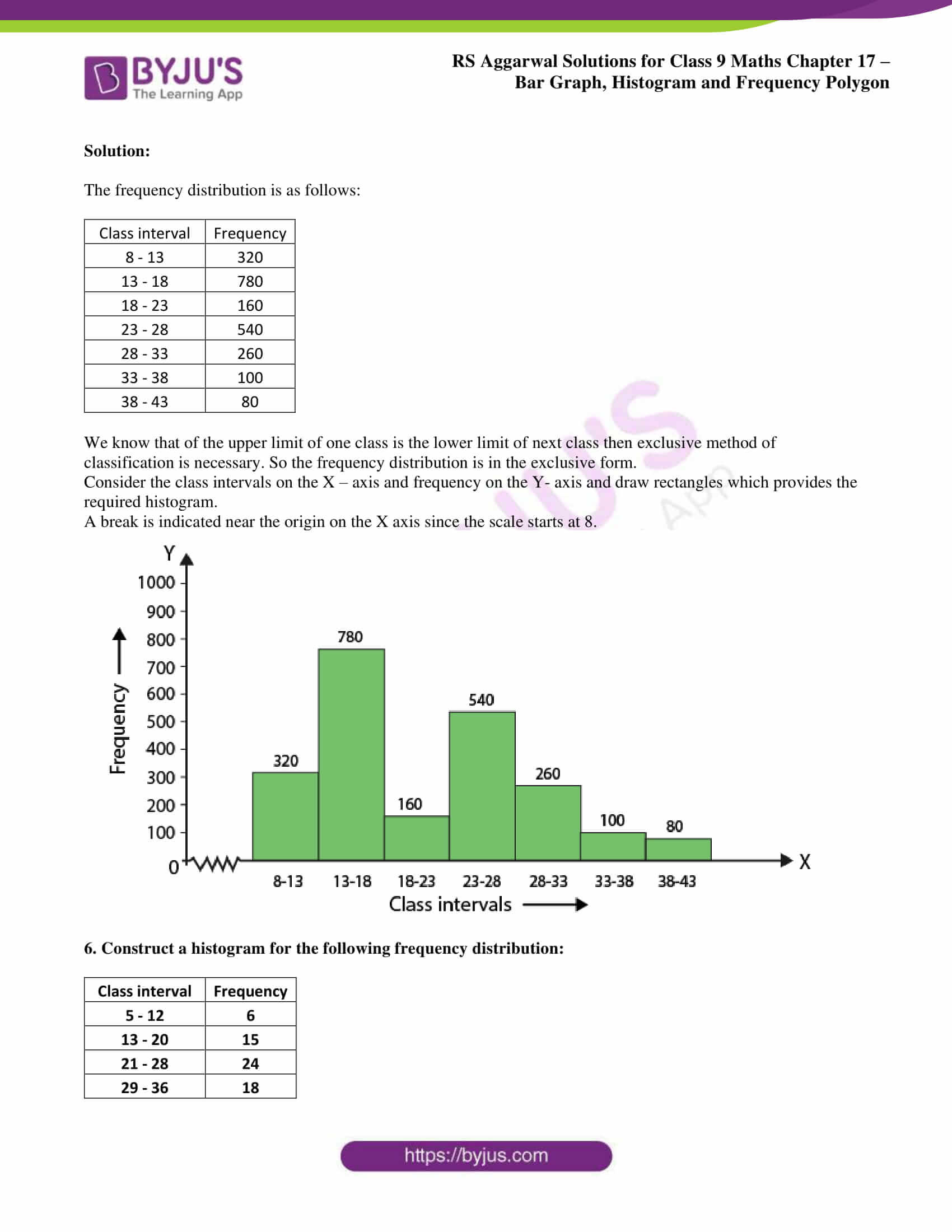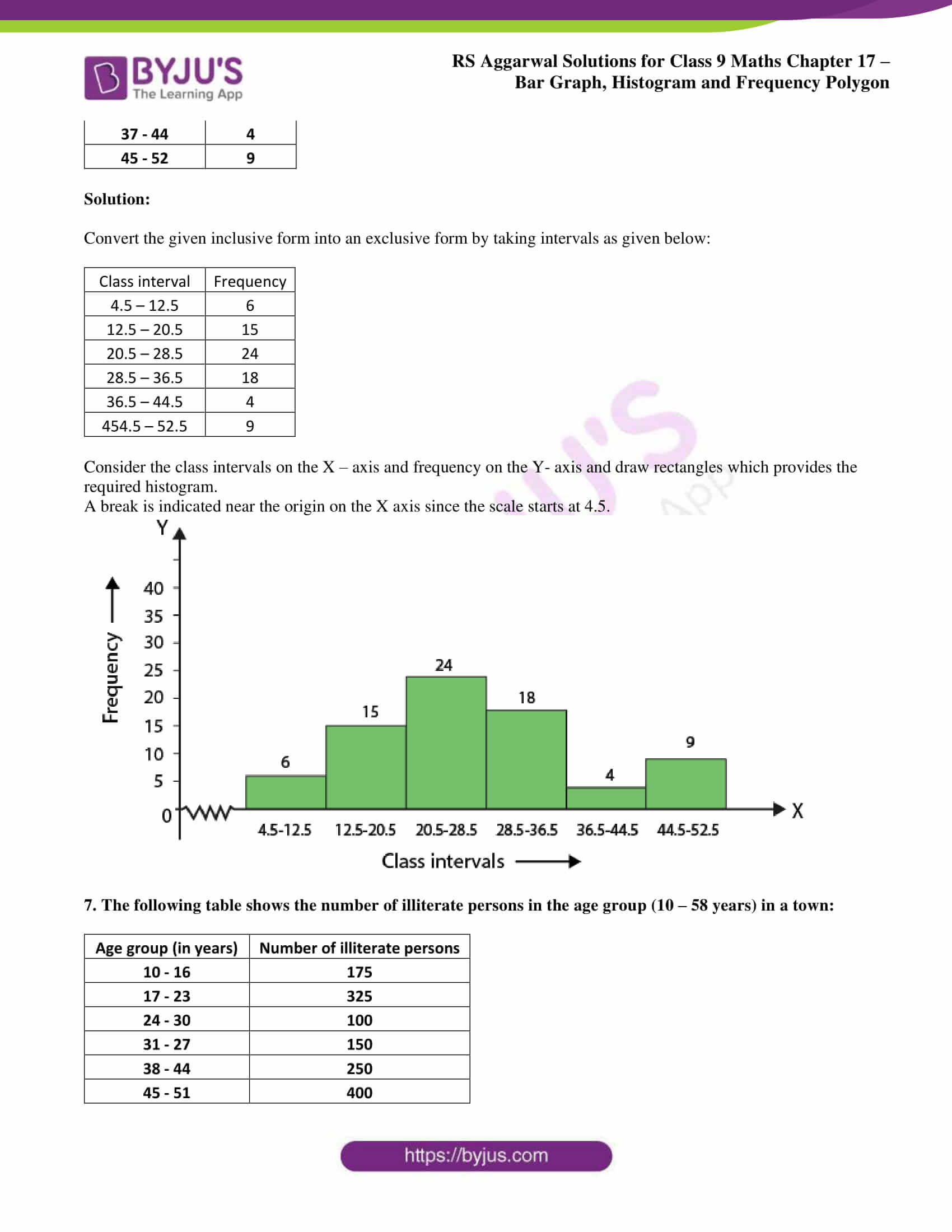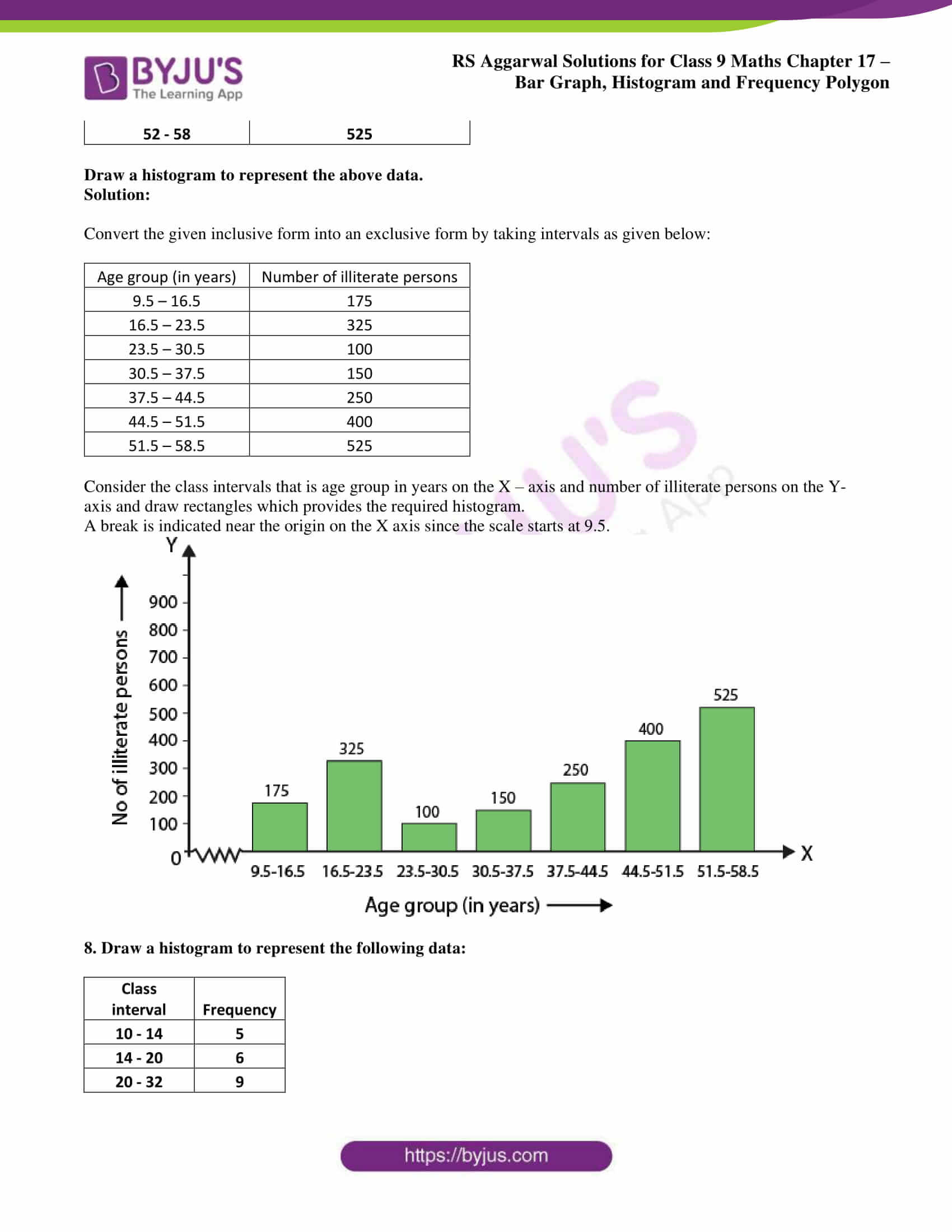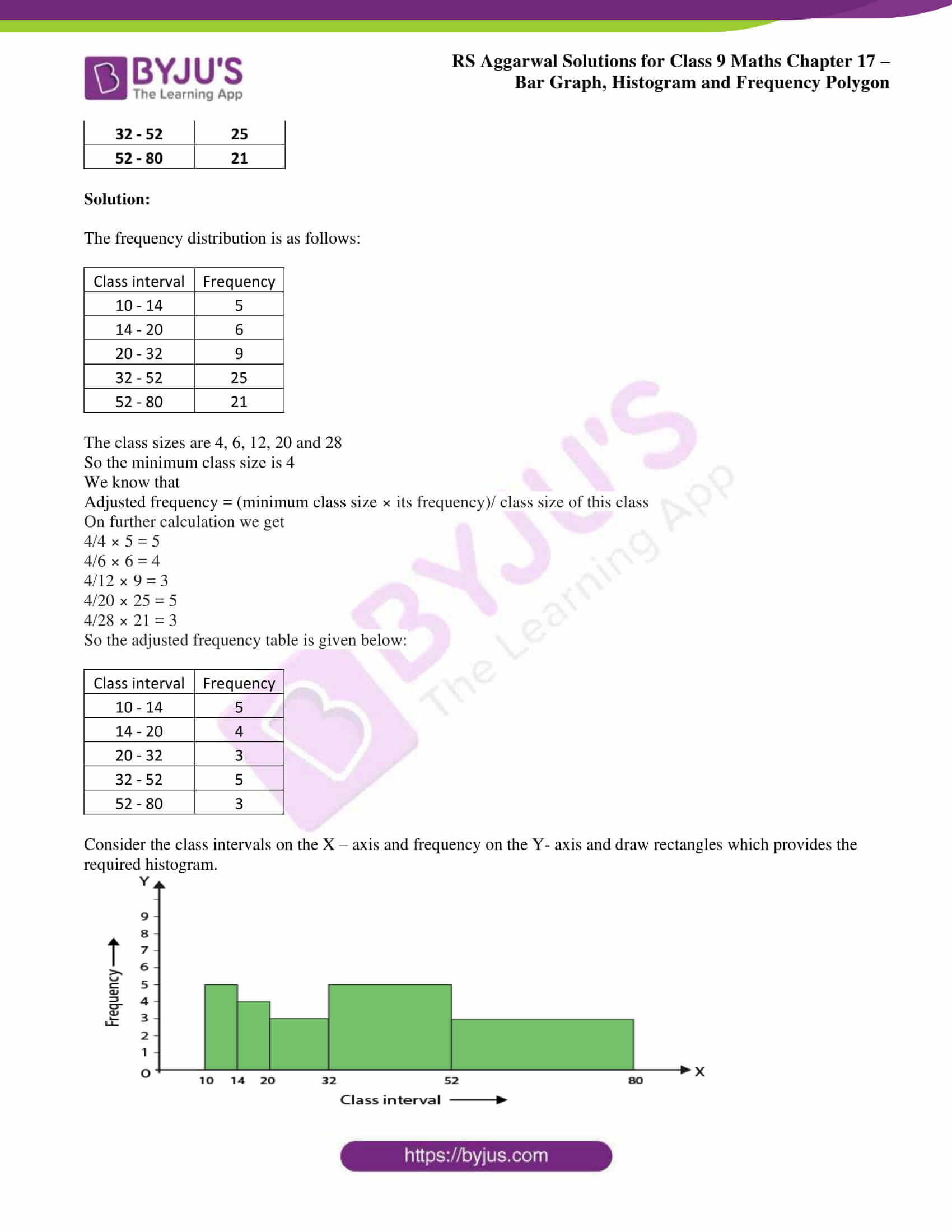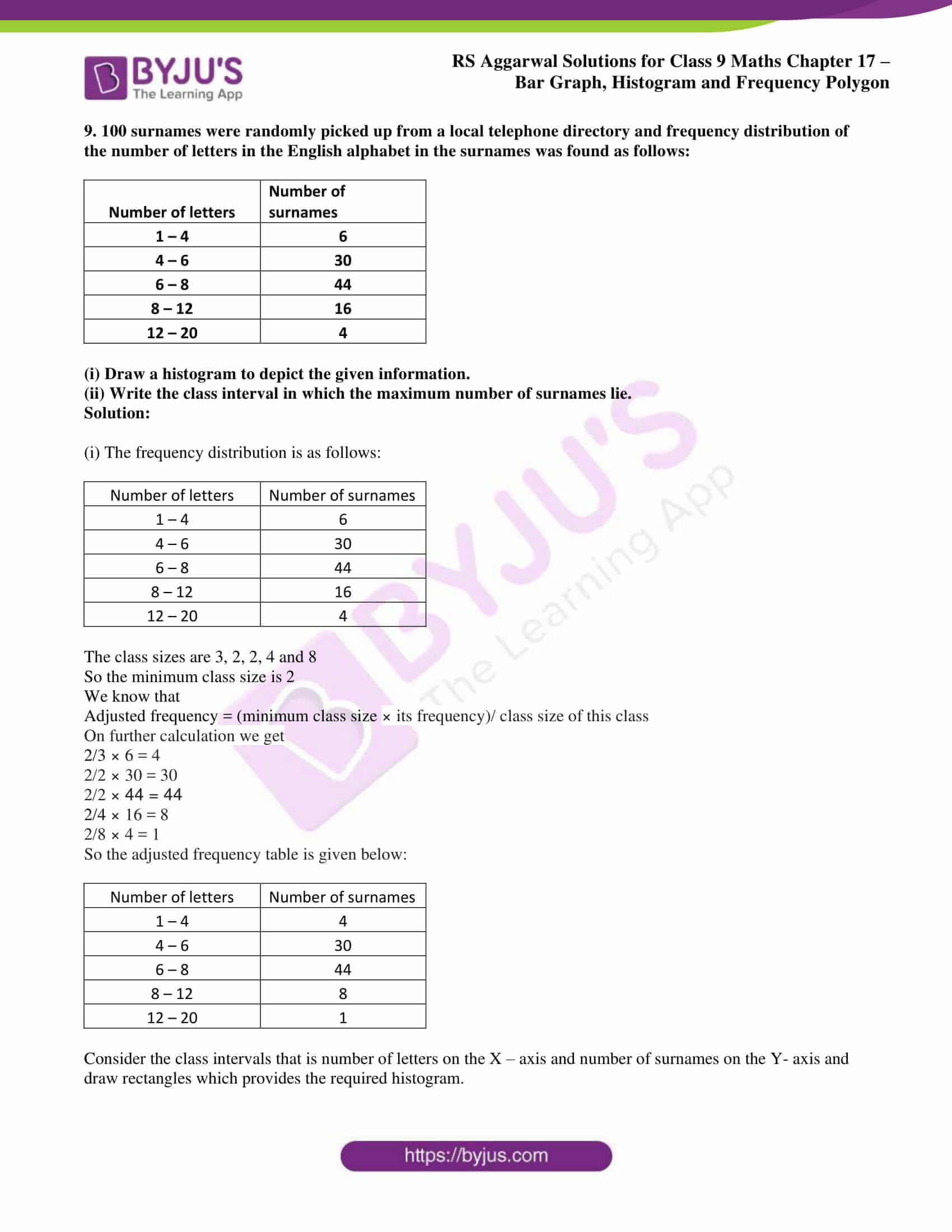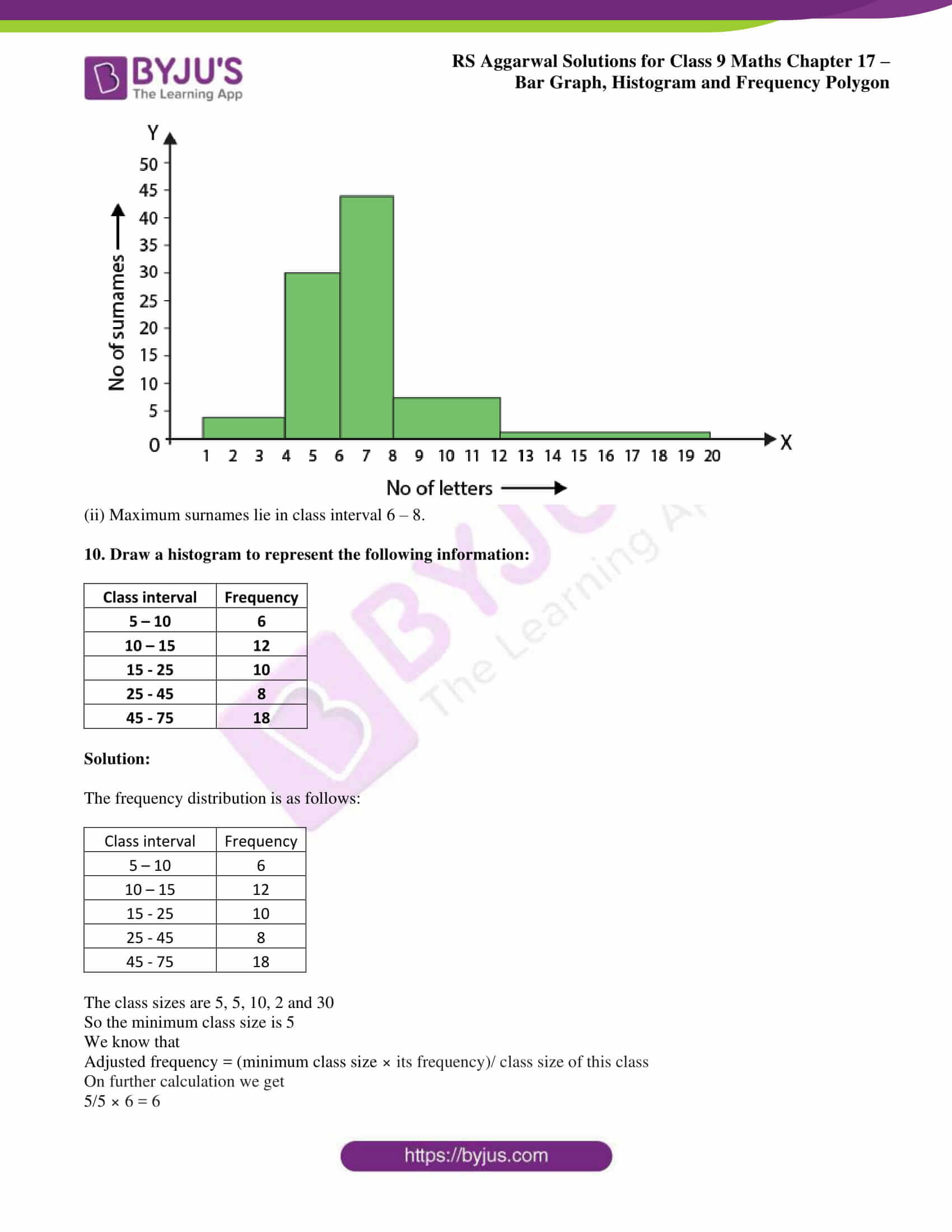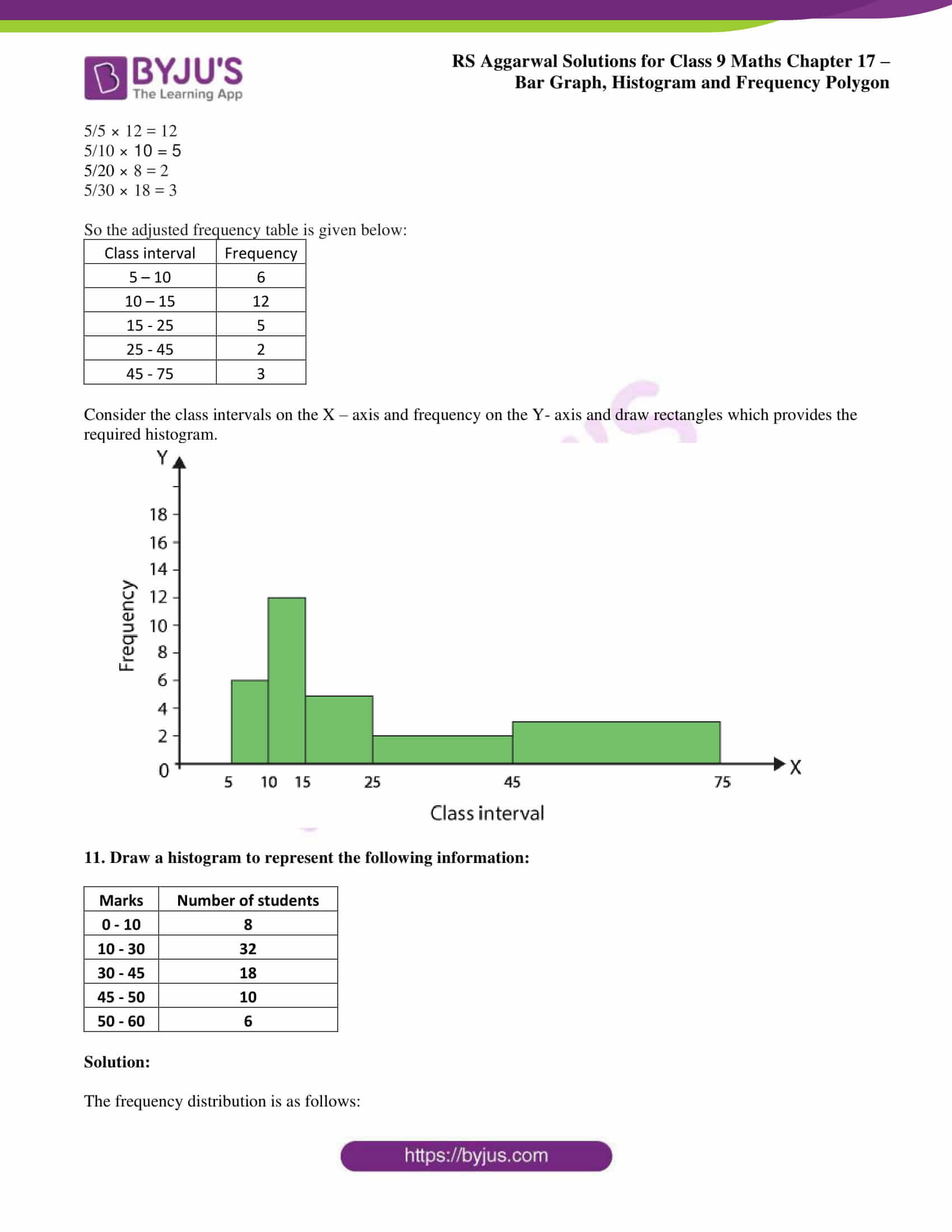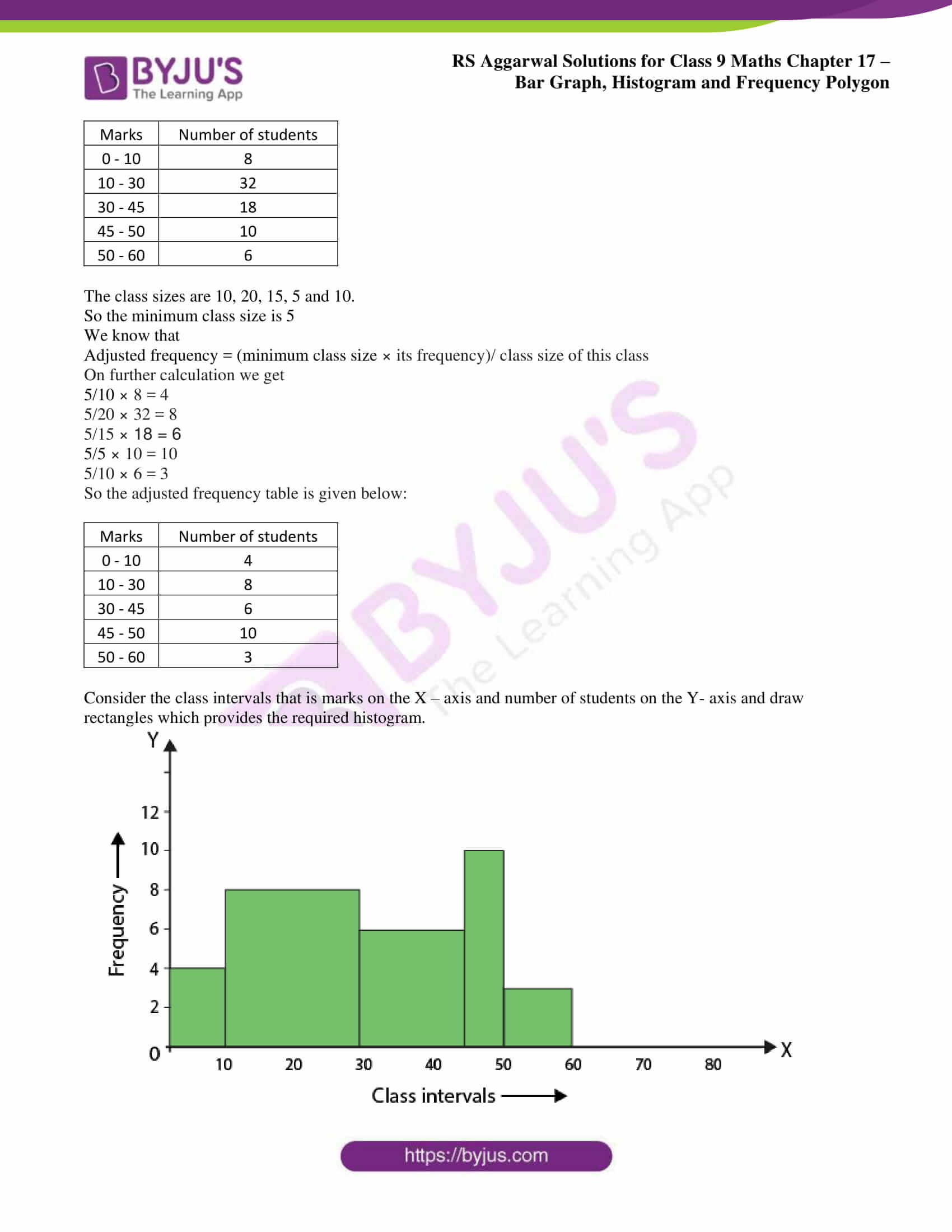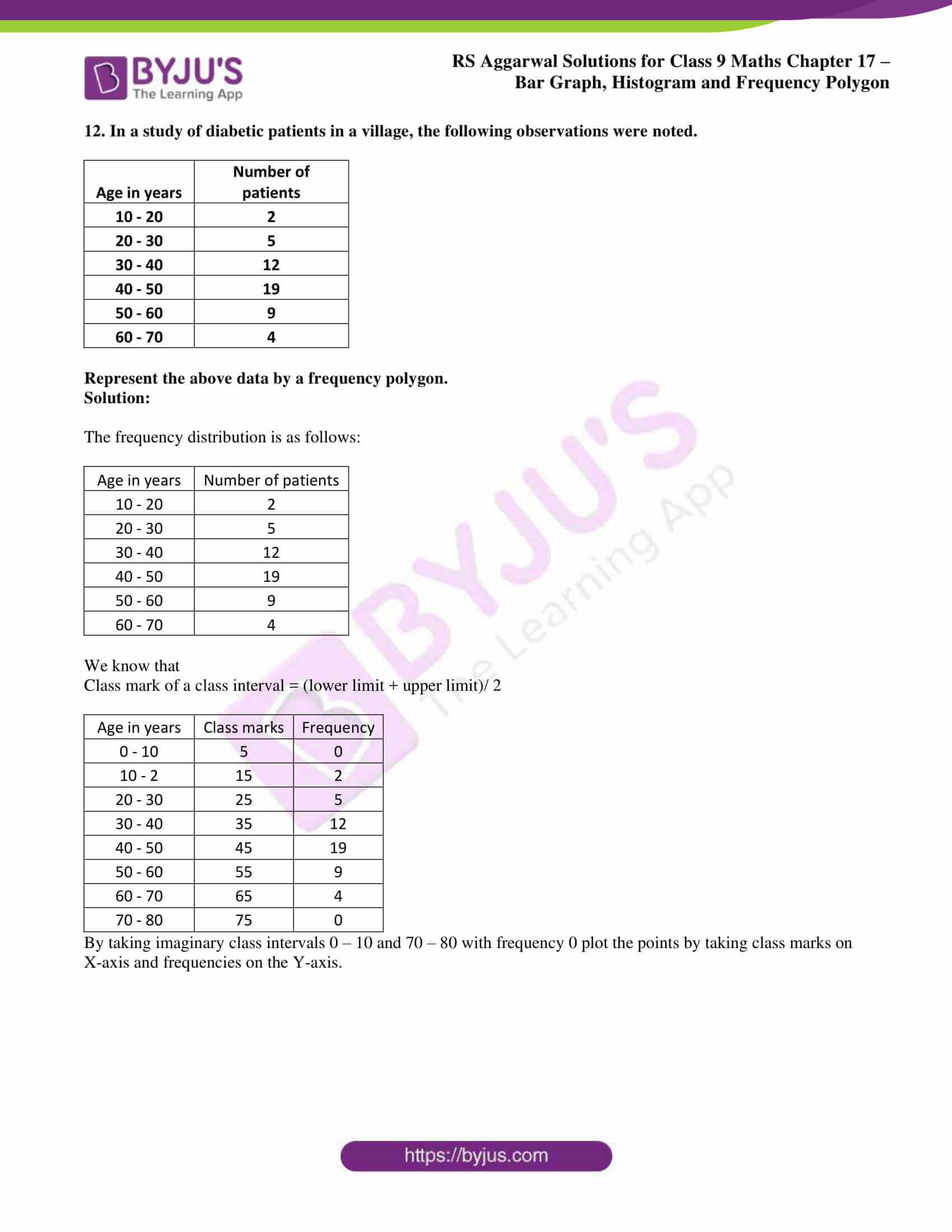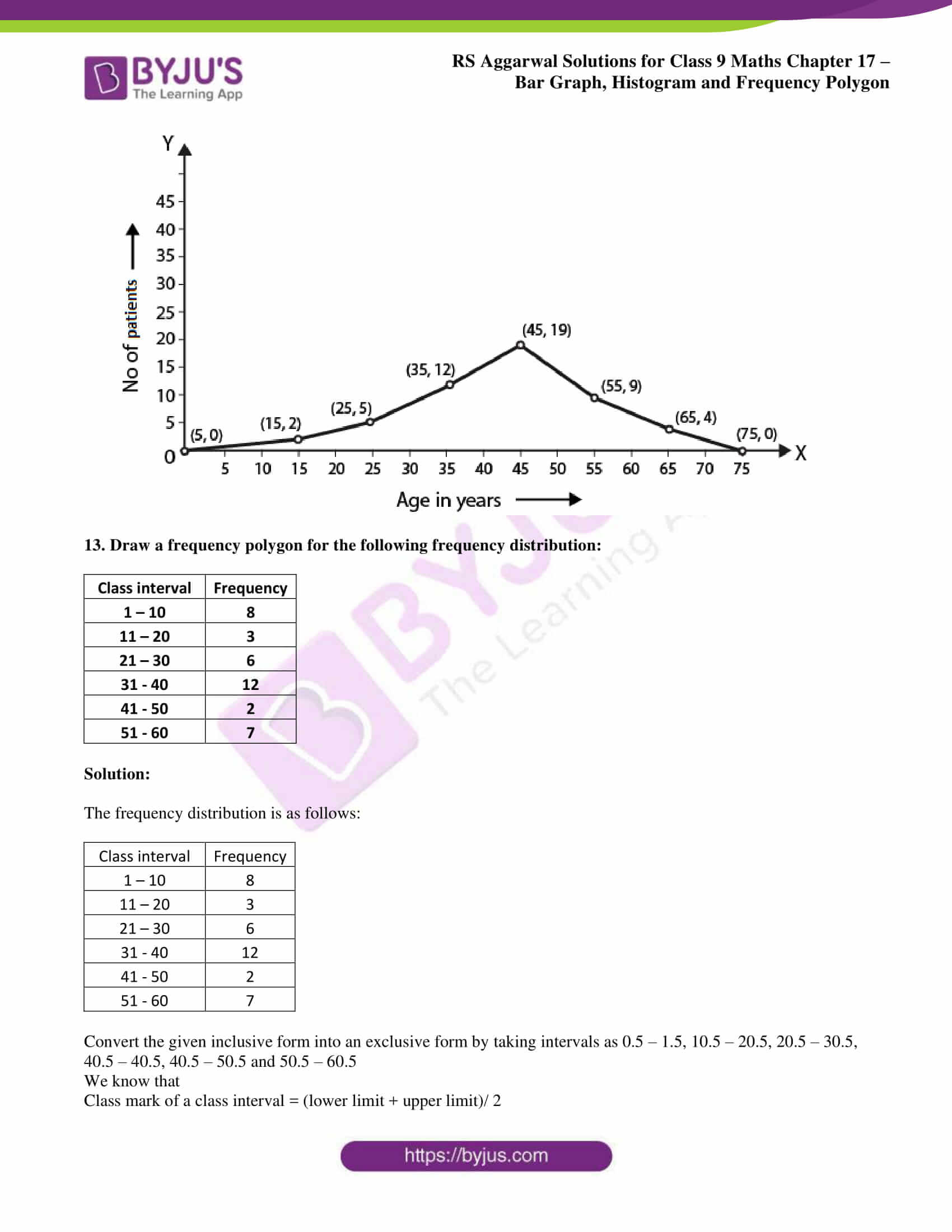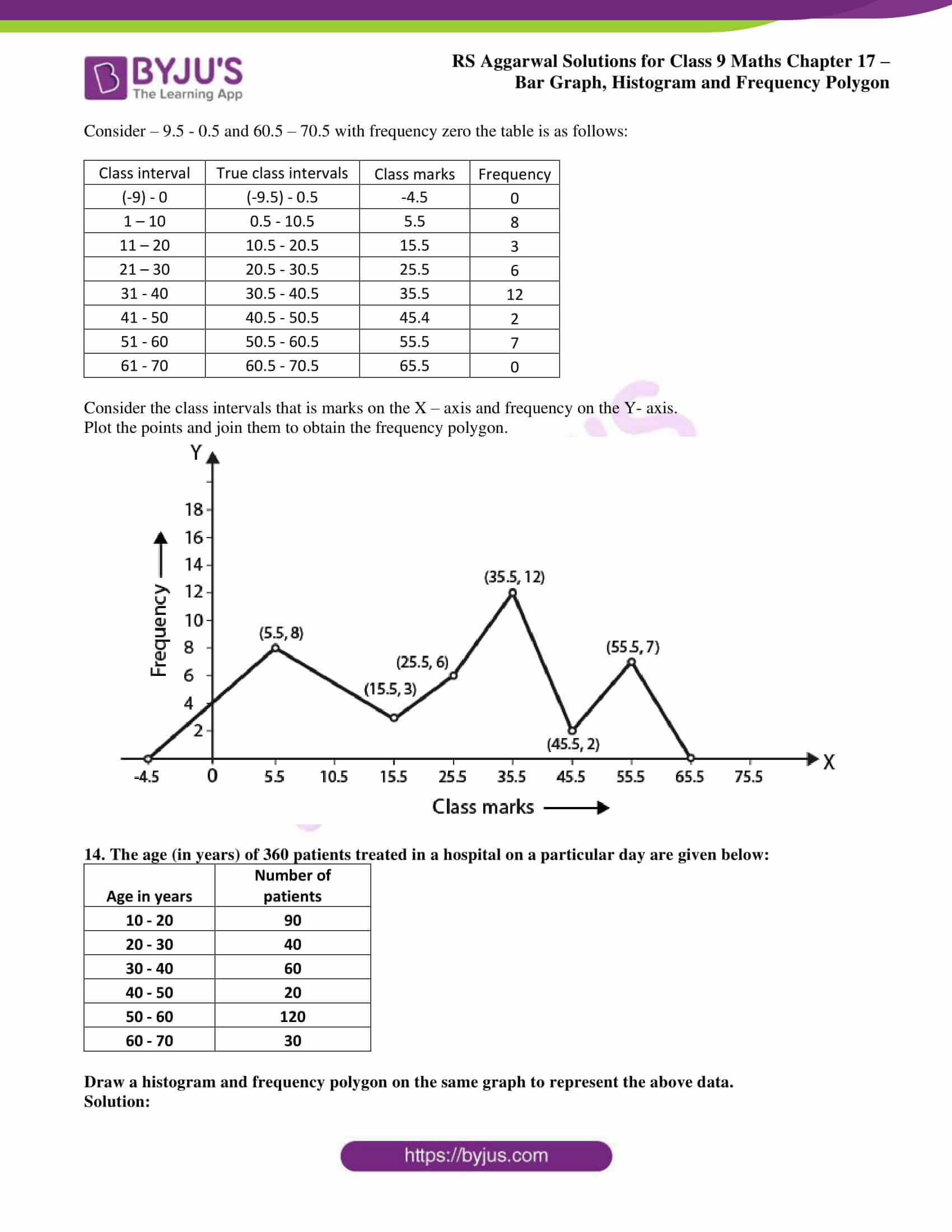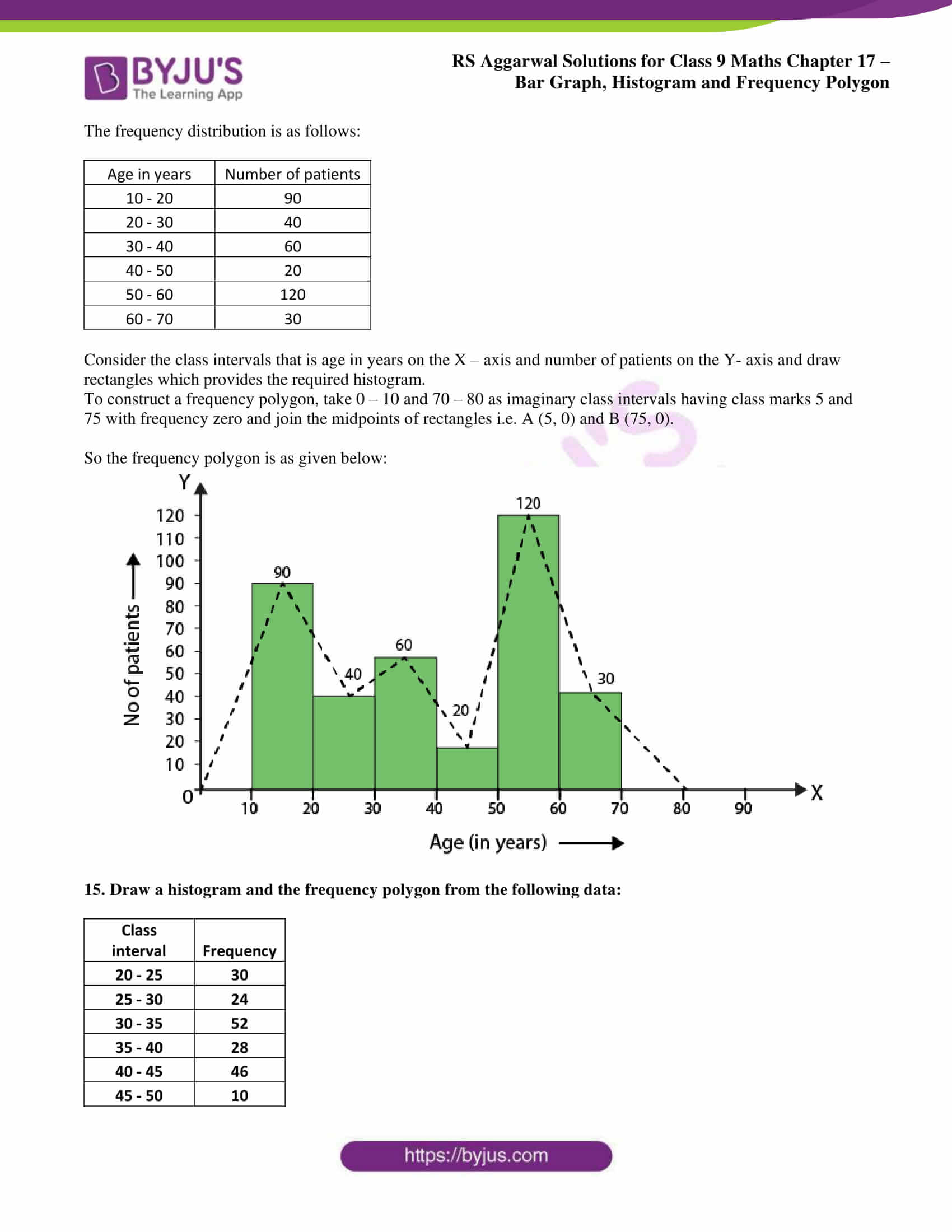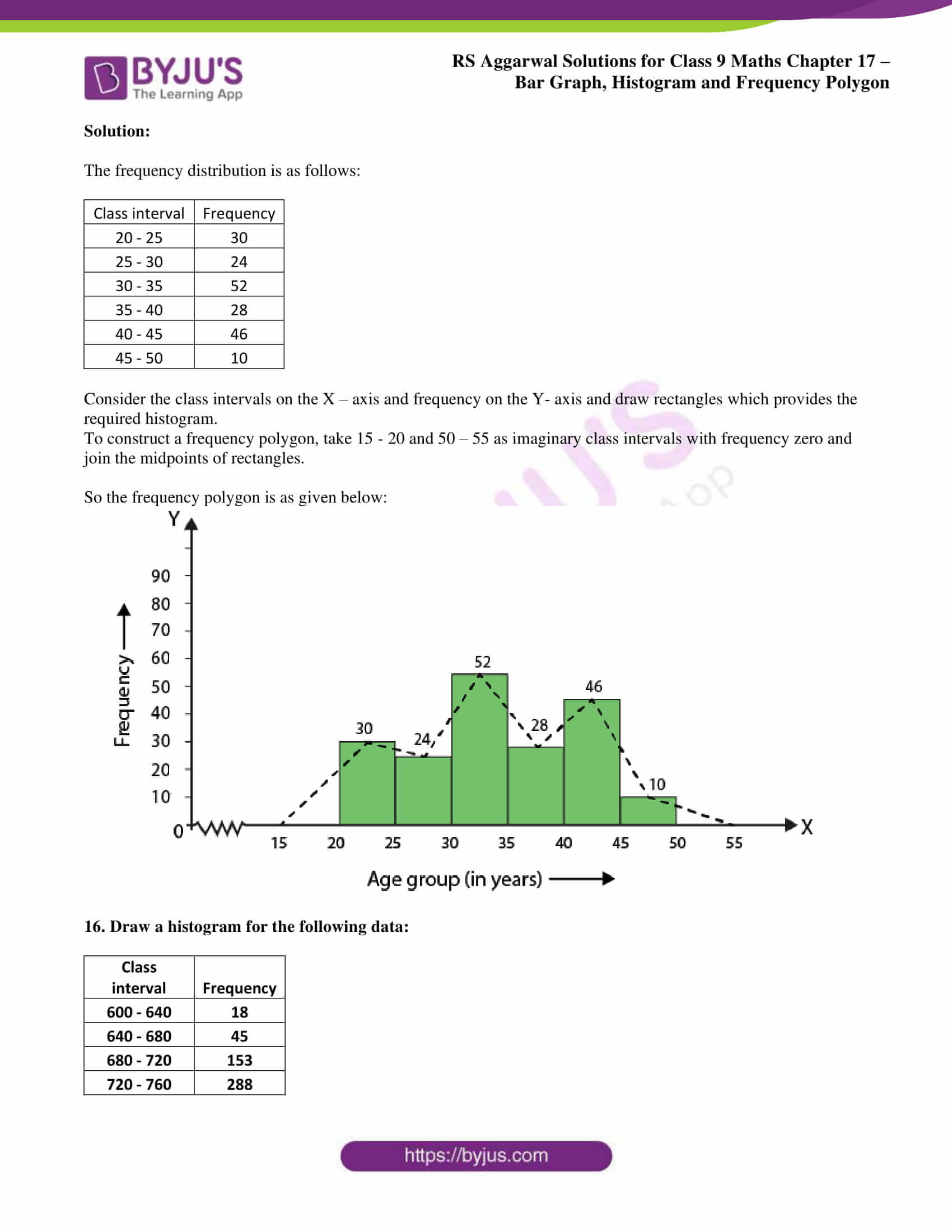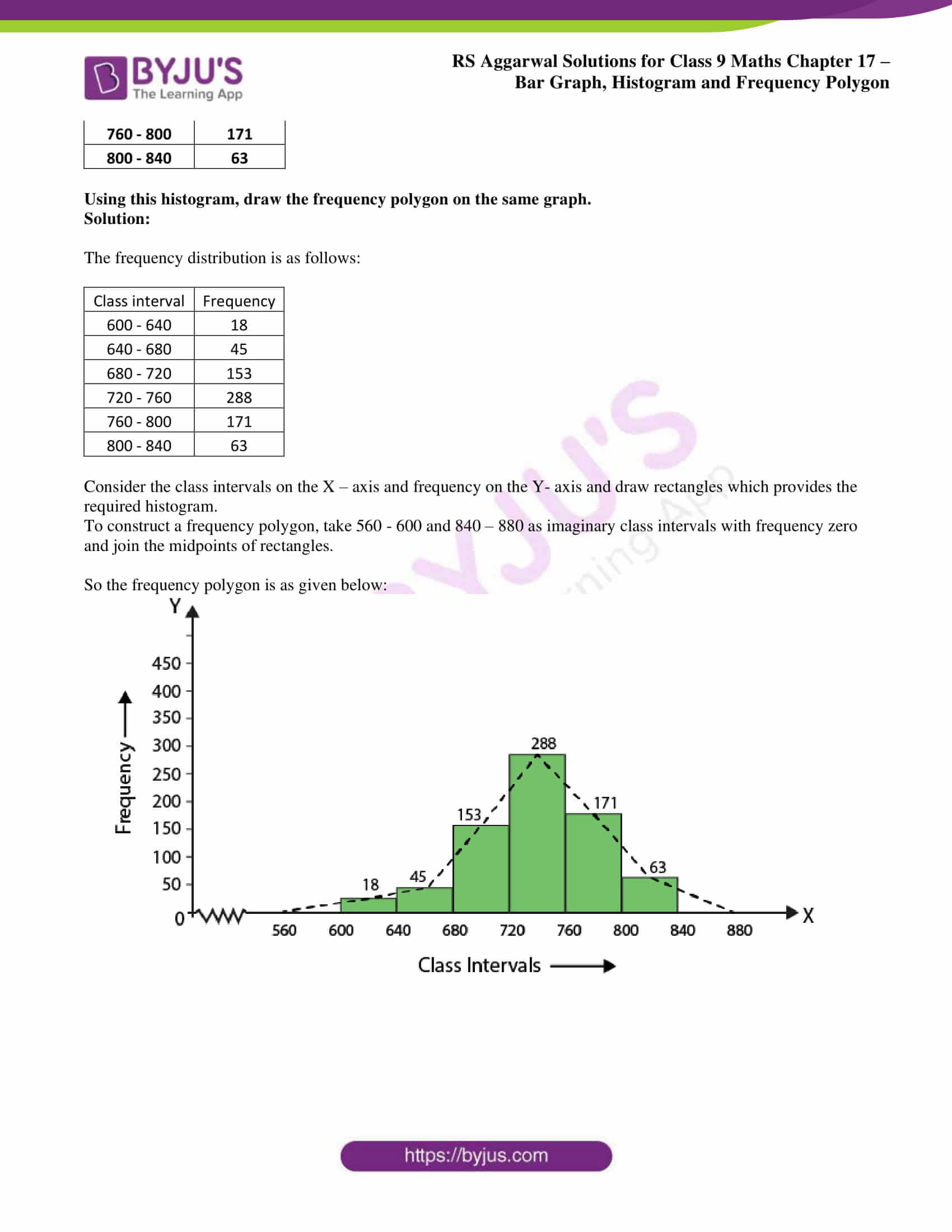## Access RS Aggarwal Solutions for Class 9 Chapter 17: Bar Graph, Histogram and Frequency Polygon Exercise 17B

### Exercise 17(B) page: 658

1. The daily wages of 50 workers in a factory are given below:

 Daily wages (in ₹) Number of workers 340 – 380 16 380 – 420 9 420 – 460 12 460 – 500 2 500 – 540 7 540 – 580 4

Construct a histogram to represent the above frequency distribution.

Solution:

The frequency distribution is as follows:

 Daily wages (in ₹) Number of workers 340 – 380 16 380 – 420 9 420 – 460 12 460 – 500 2 500 – 540 7 540 – 580 4

We know that of the upper limit of one class is the lower limit of next class then exclusive method of classification is necessary. So the frequency distribution is in the exclusive form.

Consider the class intervals that is Daily wages (in ₹) on the X – axis and number of workers on the Y- axis and draw rectangles which provides the required histogram.

A break is indicated near the origin on the X axis since the scale starts at 340.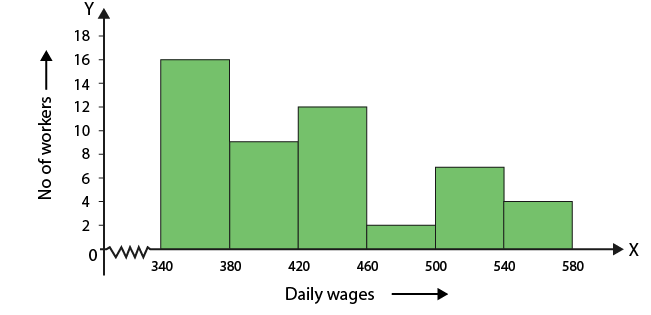2. The following table shows the average daily earnings of 40 general stores in a market, during a certain week:

 Daily earning (in rupees) Number of stores 700 – 750 6 750 – 800 9 800 – 850 2 850 – 900 7 900 – 950 11 950 – 1000 5

Draw a histogram to represent the above data.

Solution:

The frequency distribution is as follows:

 Daily earning (in rupees) Number of stores 700 – 750 6 750 – 800 9 800 – 850 2 850 – 900 7 900 – 950 11 950 – 1000 5

We know that of the upper limit of one class is the lower limit of next class then exclusive method of classification is necessary. So the frequency distribution is in the exclusive form.

Consider the class intervals that is Daily earning in rupees on the X – axis and number of stores on the Y- axis and draw rectangles which provides the required histogram.

A break is indicated near the origin on the X axis since the scale starts at 700.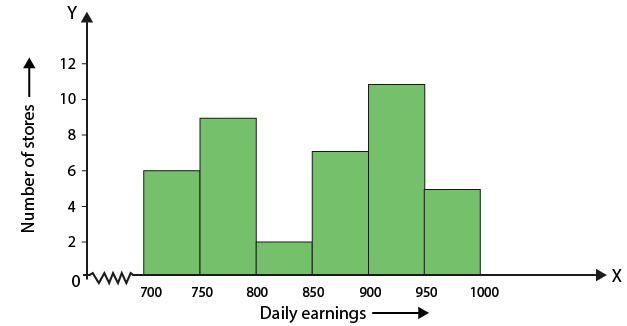3. The heights of 75 students in a school are given below:

 Height (in cm) Number of students 130 – 136 9 136 – 142 12 142 – 148 18 148 – 154 23 154 – 160 10 160 – 166 3

Draw a histogram to represent the above data.

Solution:

The frequency distribution is as follows:

 Height (in cm) Number of students 130 – 136 9 136 – 142 12 142 – 148 18 148 – 154 23 154 – 160 10 160 – 166 3

We know that of the upper limit of one class is the lower limit of next class then exclusive method of classification is necessary. So the frequency distribution is in the exclusive form.

Consider the class intervals that is height in cm on the X – axis and number of students on the Y- axis and draw rectangles which provides the required histogram.

A break is indicated near the origin on the X axis since the scale starts at 130.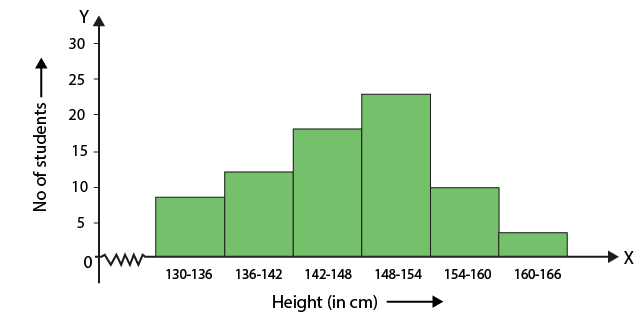4. The following table gives the lifetimes of 400 neon lamps:

 Lifetime (in hr) Number of lamps 300 – 400 14 400 – 500 56 500 – 600 60 600 – 700 86 700 – 800 74 800 – 900 62 900 – 1000 48

(i) Represent the given information with the help of a histogram.

(ii) How many lamps have a lifetime of more than 700 hours?

Solution:

(i) Histogram is given below: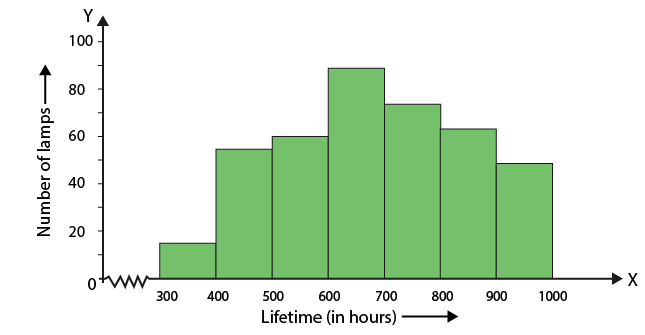(ii) Number of lamps having a lifetime of more than 700 hours = (74 + 62 + 48) = 184.

5. Draw a histogram for the frequency distribution of the following data:

 Class interval Frequency 8 – 13 320 13 – 18 780 18 – 23 160 23 – 28 540 28 – 33 260 33 – 38 100 38 – 43 80

Solution:

The frequency distribution is as follows:

 Class interval Frequency 8 – 13 320 13 – 18 780 18 – 23 160 23 – 28 540 28 – 33 260 33 – 38 100 38 – 43 80

We know that of the upper limit of one class is the lower limit of next class then exclusive method of classification is necessary. So the frequency distribution is in the exclusive form.

Consider the class intervals on the X – axis and frequency on the Y- axis and draw rectangles which provides the required histogram.

A break is indicated near the origin on the X axis since the scale starts at 8.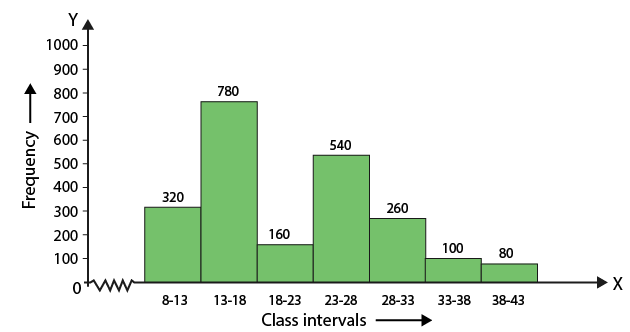6. Construct a histogram for the following frequency distribution:

 Class interval Frequency 5 – 12 6 13 – 20 15 21 – 28 24 29 – 36 18 37 – 44 4 45 – 52 9

Solution:

Convert the given inclusive form into an exclusive form by taking intervals as given below:

 Class interval Frequency 4.5 – 12.5 6 12.5 – 20.5 15 20.5 – 28.5 24 28.5 – 36.5 18 36.5 – 44.5 4 454.5 – 52.5 9

Consider the class intervals on the X – axis and frequency on the Y- axis and draw rectangles which provides the required histogram.

A break is indicated near the origin on the X axis since the scale starts at 4.5.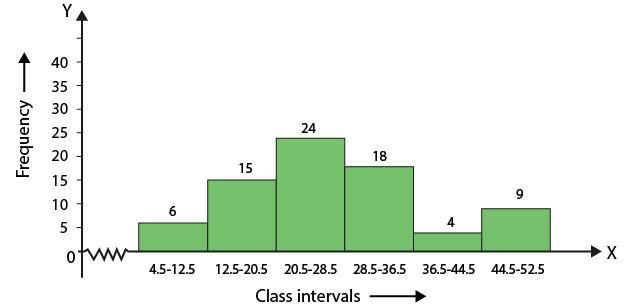7. The following table shows the number of illiterate persons in the age group (10 – 58 years) in a town:

 Age group (in years) Number of illiterate persons 10 – 16 175 17 – 23 325 24 – 30 100 31 – 27 150 38 – 44 250 45 – 51 400 52 – 58 525

Draw a histogram to represent the above data.

Solution:

Convert the given inclusive form into an exclusive form by taking intervals as given below:

 Age group (in years) Number of illiterate persons 9.5 – 16.5 175 16.5 – 23.5 325 23.5 – 30.5 100 30.5 – 37.5 150 37.5 – 44.5 250 44.5 – 51.5 400 51.5 – 58.5 525

Consider the class intervals that is age group in years on the X – axis and number of illiterate persons on the Y- axis and draw rectangles which provides the required histogram.

A break is indicated near the origin on the X axis since the scale starts at 9.5.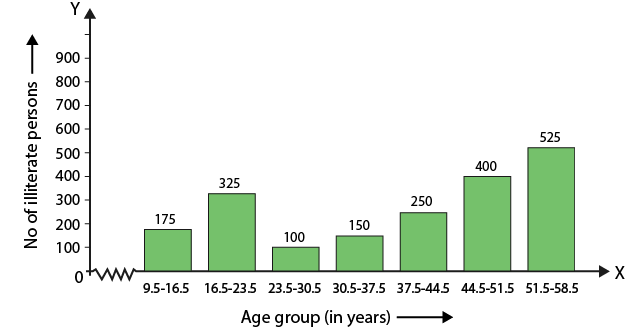8. Draw a histogram to represent the following data:

 Class interval Frequency 10 – 14 5 14 – 20 6 20 – 32 9 32 – 52 25 52 – 80 21

Solution:

The frequency distribution is as follows:

 Class interval Frequency 10 – 14 5 14 – 20 6 20 – 32 9 32 – 52 25 52 – 80 21

The class sizes are 4, 6, 12, 20 and 28

So the minimum class size is 4

We know that

Adjusted frequency = (minimum class size × its frequency)/ class size of this class

On further calculation we get

4/4 × 5 = 5

4/6 × 6 = 4

4/12 × 9 = 3

4/20 × 25 = 5

4/28 × 21 = 3

So the adjusted frequency table is given below:

 Class interval Frequency 10 – 14 5 14 – 20 4 20 – 32 3 32 – 52 5 52 – 80 3

Consider the class intervals on the X – axis and frequency on the Y- axis and draw rectangles which provides the required histogram.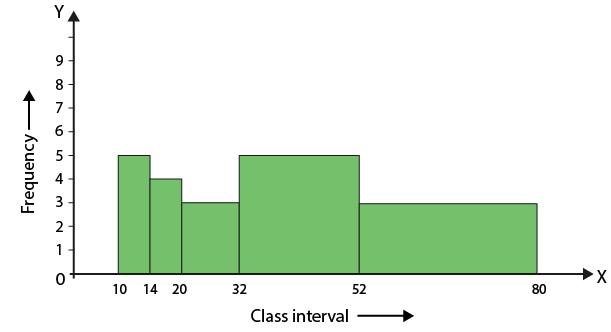9. 100 surnames were randomly picked up from a local telephone directory and frequency distribution of the number of letters in the English alphabet in the surnames was found as follows:

 Number of letters Number of surnames 1 – 4 6 4 – 6 30 6 – 8 44 8 – 12 16 12 – 20 4

(i) Draw a histogram to depict the given information.

(ii) Write the class interval in which the maximum number of surnames lie.

Solution:

(i) The frequency distribution is as follows:

 Number of letters Number of surnames 1 – 4 6 4 – 6 30 6 – 8 44 8 – 12 16 12 – 20 4

The class sizes are 3, 2, 2, 4 and 8

So the minimum class size is 2

We know that

Adjusted frequency = (minimum class size × its frequency)/ class size of this class

On further calculation we get

2/3 × 6 = 4

2/2 × 30 = 30

2/2 × 44 = 44

2/4 × 16 = 8

2/8 × 4 = 1

So the adjusted frequency table is given below:

 Number of letters Number of surnames 1 – 4 4 4 – 6 30 6 – 8 44 8 – 12 8 12 – 20 1

Consider the class intervals that is number of letters on the X – axis and number of surnames on the Y- axis and draw rectangles which provides the required histogram.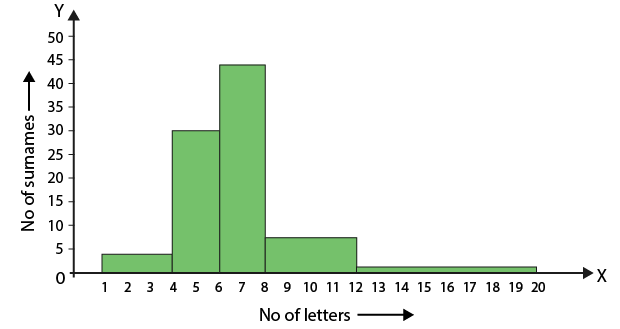(ii) Maximum surnames lie in class interval 6 – 8.

10. Draw a histogram to represent the following information:

 Class interval Frequency 5 – 10 6 10 – 15 12 15 – 25 10 25 – 45 8 45 – 75 18

Solution:

The frequency distribution is as follows:

 Class interval Frequency 5 – 10 6 10 – 15 12 15 – 25 10 25 – 45 8 45 – 75 18

The class sizes are 5, 5, 10, 2 and 30

So the minimum class size is 5

We know that

Adjusted frequency = (minimum class size × its frequency)/ class size of this class

On further calculation we get

5/5 × 6 = 6

5/5 × 12 = 12

5/10 × 10 = 5

5/20 × 8 = 2

5/30 × 18 = 3

So the adjusted frequency table is given below:

 Class interval Frequency 5 – 10 6 10 – 15 12 15 – 25 5 25 – 45 2 45 – 75 3

Consider the class intervals on the X – axis and frequency on the Y- axis and draw rectangles which provides the required histogram.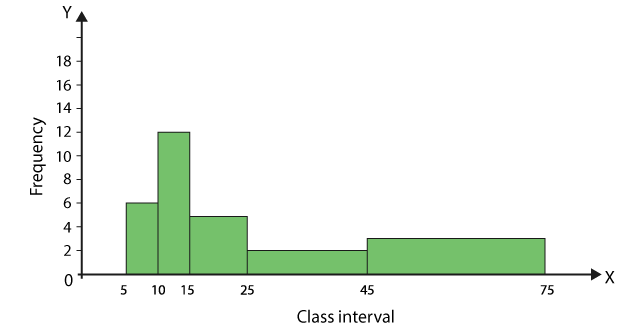11. Draw a histogram to represent the following information:

 Marks Number of students 0 – 10 8 10 – 30 32 30 – 45 18 45 – 50 10 50 – 60 6

Solution:

The frequency distribution is as follows:

 Marks Number of students 0 – 10 8 10 – 30 32 30 – 45 18 45 – 50 10 50 – 60 6

The class sizes are 10, 20, 15, 5 and 10.

So the minimum class size is 5

We know that

Adjusted frequency = (minimum class size × its frequency)/ class size of this class

On further calculation we get

5/10 × 8 = 4

5/20 × 32 = 8

5/15 × 18 = 6

5/5 × 10 = 10

5/10 × 6 = 3

So the adjusted frequency table is given below:

 Marks Number of students 0 – 10 4 10 – 30 8 30 – 45 6 45 – 50 10 50 – 60 3

Consider the class intervals that is marks on the X – axis and number of students on the Y- axis and draw rectangles which provides the required histogram.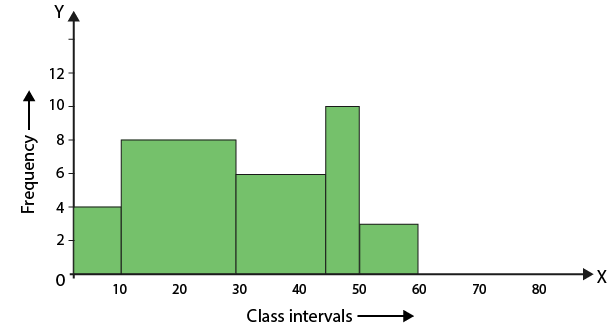12. In a study of diabetic patients in a village, the following observations were noted.

 Age in years Number of patients 10 – 20 2 20 – 30 5 30 – 40 12 40 – 50 19 50 – 60 9 60 – 70 4

Represent the above data by a frequency polygon.

Solution:

The frequency distribution is as follows:

 Age in years Number of patients 10 – 20 2 20 – 30 5 30 – 40 12 40 – 50 19 50 – 60 9 60 – 70 4

We know that

Class mark of a class interval = (lower limit + upper limit)/ 2

 Age in years Class marks Frequency 0 – 10 5 0 10 – 2 15 2 20 – 30 25 5 30 – 40 35 12 40 – 50 45 19 50 – 60 55 9 60 – 70 65 4 70 – 80 75 0

By taking imaginary class intervals 0 – 10 and 70 – 80 with frequency 0 plot the points by taking class marks on X-axis and frequencies on the Y-axis.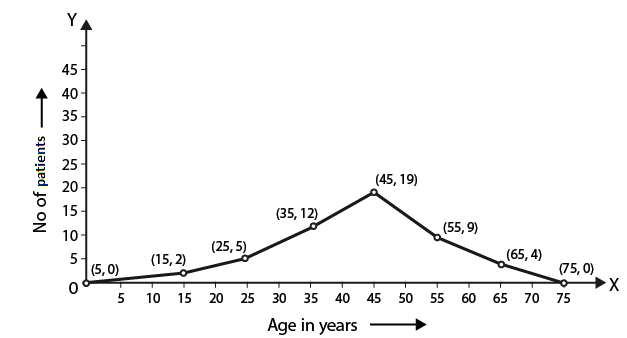13. Draw a frequency polygon for the following frequency distribution:

 Class interval Frequency 1 – 10 8 11 – 20 3 21 – 30 6 31 – 40 12 41 – 50 2 51 – 60 7

Solution:

The frequency distribution is as follows:

 Class interval Frequency 1 – 10 8 11 – 20 3 21 – 30 6 31 – 40 12 41 – 50 2 51 – 60 7

Convert the given inclusive form into an exclusive form by taking intervals as 0.5 – 1.5, 10.5 – 20.5, 20.5 – 30.5, 40.5 – 40.5, 40.5 – 50.5 and 50.5 – 60.5

We know that

Class mark of a class interval = (lower limit + upper limit)/ 2

Consider – 9.5 – 0.5 and 60.5 – 70.5 with frequency zero the table is as follows:

 Class interval True class intervals Class marks Frequency (-9) – 0 (-9.5) – 0.5 -4.5 0 1 – 10 0.5 – 10.5 5.5 8 11 – 20 10.5 – 20.5 15.5 3 21 – 30 20.5 – 30.5 25.5 6 31 – 40 30.5 – 40.5 35.5 12 41 – 50 40.5 – 50.5 45.4 2 51 – 60 50.5 – 60.5 55.5 7 61 – 70 60.5 – 70.5 65.5 0

Consider the class intervals that is marks on the X – axis and frequency on the Y- axis.

Plot the points and join them to obtain the frequency polygon.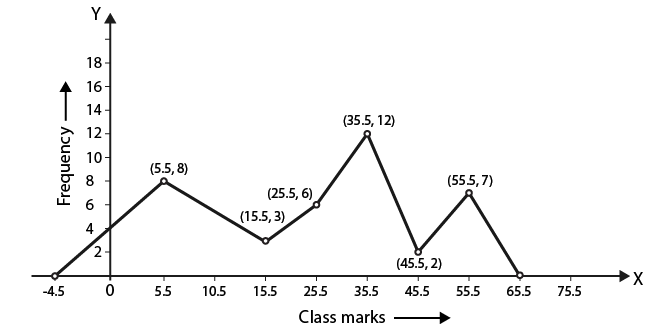14. The age (in years) of 360 patients treated in a hospital on a particular day are given below:

 Age in years Number of patients 10 – 20 90 20 – 30 40 30 – 40 60 40 – 50 20 50 – 60 120 60 – 70 30

Draw a histogram and frequency polygon on the same graph to represent the above data.

Solution:

The frequency distribution is as follows:

 Age in years Number of patients 10 – 20 90 20 – 30 40 30 – 40 60 40 – 50 20 50 – 60 120 60 – 70 30

Consider the class intervals that is age in years on the X – axis and number of patients on the Y- axis and draw rectangles which provides the required histogram.

To construct a frequency polygon, take 0 – 10 and 70 – 80 as imaginary class intervals having class marks 5 and 75 with frequency zero and join the midpoints of rectangles i.e. A (5, 0) and B (75, 0).

So the frequency polygon is as given below: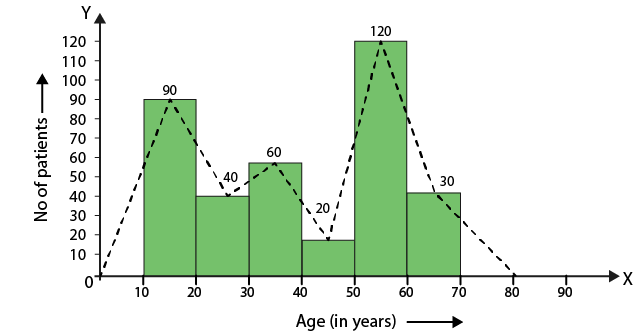15. Draw a histogram and the frequency polygon from the following data:

 Class interval Frequency 20 – 25 30 25 – 30 24 30 – 35 52 35 – 40 28 40 – 45 46 45 – 50 10

Solution:

The frequency distribution is as follows:

 Class interval Frequency 20 – 25 30 25 – 30 24 30 – 35 52 35 – 40 28 40 – 45 46 45 – 50 10

Consider the class intervals on the X – axis and frequency on the Y- axis and draw rectangles which provides the required histogram.

To construct a frequency polygon, take 15 – 20 and 50 – 55 as imaginary class intervals with frequency zero and join the midpoints of rectangles.

So the frequency polygon is as given below: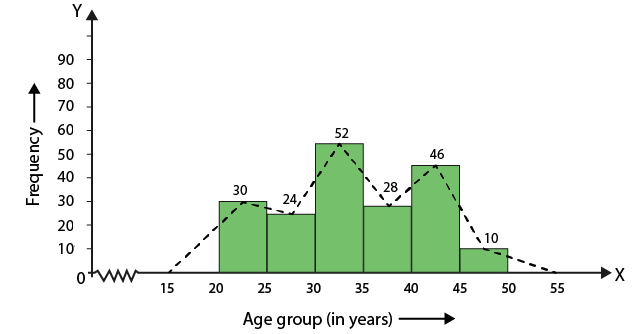16. Draw a histogram for the following data:

 Class interval Frequency 600 – 640 18 640 – 680 45 680 – 720 153 720 – 760 288 760 – 800 171 800 – 840 63

Using this histogram, draw the frequency polygon on the same graph.

Solution:

The frequency distribution is as follows:

 Class interval Frequency 600 – 640 18 640 – 680 45 680 – 720 153 720 – 760 288 760 – 800 171 800 – 840 63

Consider the class intervals on the X – axis and frequency on the Y- axis and draw rectangles which provides the required histogram.

To construct a frequency polygon, take 560 – 600 and 840 – 880 as imaginary class intervals with frequency zero and join the midpoints of rectangles.

So the frequency polygon is as given below: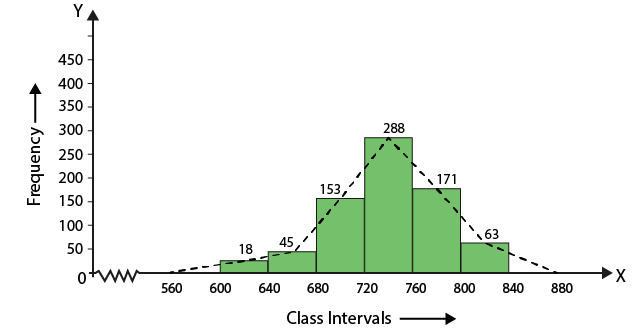### Access other exercise solutions of Class 9 Maths Chapter 17: Bar Graph, Histogram and Frequency Polygon

Exercise 17A Solutions 12 Questions

### RS Aggarwal Solutions Class 9 Maths Chapter 17 – Bar Graph, Histogram and Frequency Polygon Exercise 17B

RS Aggarwal Solutions Class 9 Maths Chapter 17 Bar Graph, Histogram and Frequency Polygon Exercise 17B explains the methods used in drawing a histogram and frequency polygon for the exercise problems.

### Key features of RS Aggarwal Solutions for Class 9 Maths Chapter 17: Bar Graph, Histogram and Frequency Polygon Exercise 17B

• Solving the examples and exercise wise problems improve the speed in answering questions in the board exam.
• The problems in RS Aggarwal textbook are based on the latest CBSE syllabus and guidelines.
• The students by solving huge numbers of problems can understand which concepts require more importance based on weightage.
• This exercise helps students with the various methods which can be used in drawing a frequency polygon and histogram.